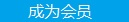您可以捐助，支持我们的公益事业。 1元 10元 50元 认证码：必填求知 文章 文库 Lib 视频 Code iProcess 课程 认证 咨询 工具 火云堂 讲座吧 成长之路要资料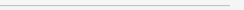订阅捐助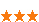2648 次浏览     评价： 好 中 差
2018-1-10

 编辑推荐: 本文来自于csdn，这个指南主要基于Denny Britz所做的工作，他深入地探索了机器人开发中深度学习技术的利用。 文章中包含代码片段和Github仓，好好利用！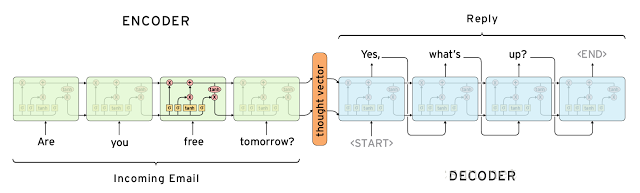“开放领域 ：可以提出一个关于任何主题的问题，并期待相关的回应，这很难实现。考虑一下，如果就抵押贷款再融资问题进行交谈的话，实际上你可以问任何事情“ ―― 马克・克拉克

“封闭领域 ：可以问一些关于特定主题的有限的问题，更容易实现。比如，迈阿密天气怎么样？“

“Square 1迈出了一个聊天机器人的可喜的第一步，它表明了可能不需要智能机器的复杂性，也可以提供商业和用户价值。

”Square 2使用了可以生成响应的智能机器技术。 生成的响应允许Chatbot处理常见问题和一些不可预见的情况，而这些情况没有预定义的响应。 智能机器可以处理更长的对话并且看起来更像人。 但是生成式响应增加了系统的复杂性，而且往往是增加了很多的复杂性。（前）百度首席科学家Andrew Ng 最近接受采访时说：

UBUNTU DIALOG CORPUS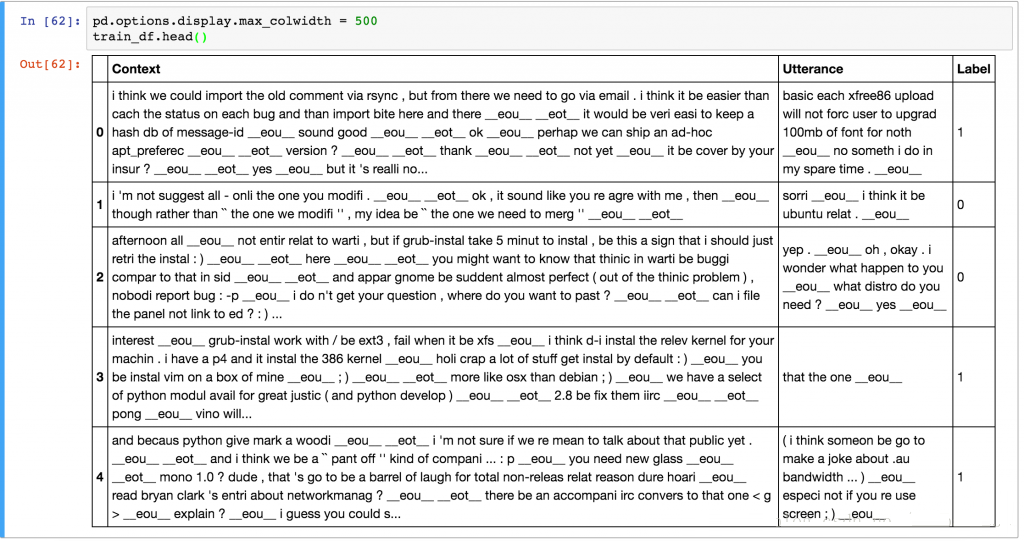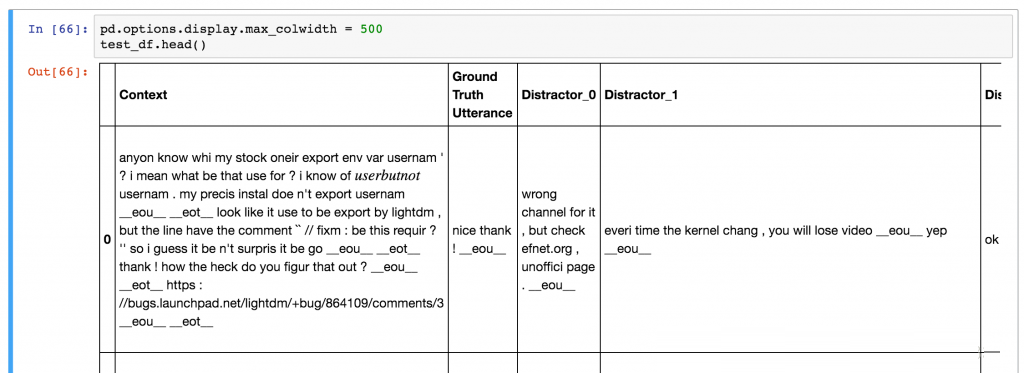def evaluate_recall(y, y_test, k=1): num_examples = float(len(y)) num_correct = 0 for predictions, label in zip(y, y_test): if label in predictions[:k]: num_correct += 1 return num_correct/num_examples

 # Random Predictor def predict_random(context, utterances): return np.random.choice(len(utterances), 10, replace=False) # Evaluate Random predictor y_random = [predict_random(test_df.Context[x], test_df.iloc[x,1:].values) for x in range(len(test_df))] y_test = np.zeros(len(y_random)) for n in [1, 2, 5, 10]: print(“Recall @ ({}, 10): {:g}”.format(n, evaluate_recall(y_random, y_test, n)))

 Recall @ (1, 10): 0.0937632 Recall @ (2, 10): 0.194503 Recall @ (5, 10): 0.49297 Recall @ (10, 10): 1

 class TFIDFPredictor: def __init__(self): self.vectorizer = TfidfVectorizer() def train(self, data): self.vectorizer.fit(np.append(data.Context.values, data.Utterance.values)) def predict(self, context, utterances): # Convert context and utterances into tfidf vector vector_context = self.vectorizer.transform([context]) vector_doc = self.vectorizer.transform(utterances) # The dot product measures the similarity of the resulting vectors result = np.dot(vector_doc, vector_context.T).todense() result = np.asarray(result).flatten() # Sort by top results and return the indices in descending order return np.argsort(result, axis=0)[::-1] # Evaluate TFIDF predictor pred = TFIDFPredictor() pred.train(train_df) y = [pred.predict(test_df.Context[x], test_df.iloc[x,1:].values) for x in range(len(test_df))] for n in [1, 2, 5, 10]: print(“Recall @ ({}, 10): {:g}”.format(n, evaluate_recall(y, y_test, n)))

 Recall @ (1, 10): 0.495032 Recall @ (2, 10): 0.596882 Recall @ (5, 10): 0.766121 Recall @ (10, 10): 1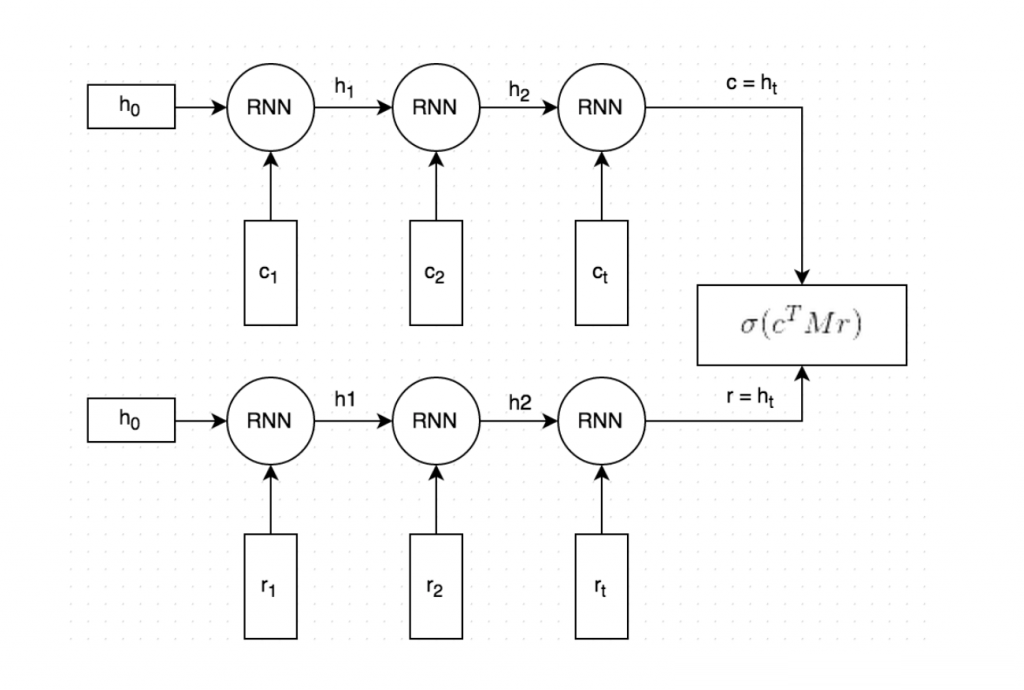context：表示上下文文本的词序列，例如[231,2190,737,0,912]

context_len：上下文的长度，例如上面例子中的5

utterance：表示话语（响应）的一系列单词id

utterance_len：话语的长度

label：标签，在训练数据中才有。 0或1。

distractor_ [N]：仅在测试/验证数据中。 N的范围从0到8.代表干扰项的词序列id。

distractor_ [N] _len：仅在测试/验证数据中。 N的范围是从0到8.发音的长度。

 def input_fn(): # TODO Load and preprocess data here return batched_features, labels

 def create_input_fn(mode, input_files, batch_size, num_epochs=None): def input_fn(): # TODO Load and preprocess data here return batched_features, labels return input_fn

 def create_evaluation_metrics(): eval_metrics = {} for k in [1, 2, 5, 10]: eval_metrics[“recall_at_%d” % k] = functools.partial( tf.contrib.metrics.streaming_sparse_recall_at_k, k=k) return eval_metricsestimator = tf.contrib.learn.Estimator( model_fn=model_fn, model_dir=MODEL_DIR, config=tf.contrib.learn.RunConfig()) input_fn_train = udc_inputs.create_input_fn( mode=tf.contrib.learn.ModeKeys.TRAIN, input_files=[TRAIN_FILE], batch_size=hparams.batch_size) input_fn_eval = udc_inputs.create_input_fn( mode=tf.contrib.learn.ModeKeys.EVAL, input_files=[VALIDATION_FILE], batch_size=hparams.eval_batch_size, num_epochs=1) eval_metrics = udc_metrics.create_evaluation_metrics() # We need to subclass theis manually for now. The next TF version will # have support ValidationMonitors with metrics built-in. # It’s already on the master branch. class EvaluationMonitor(tf.contrib.learn.monitors.EveryN): def every_n_step_end(self, step, outputs): self._estimator.evaluate( input_fn=input_fn_eval, metrics=eval_metrics, steps=None) eval_monitor = EvaluationMonitor(every_n_steps=FLAGS.eval_every) estimator.fit(input_fn=input_fn_train, steps=None, monitors=[eval_monitor])

 def dual_encoder_model( hparams, mode, context, context_len, utterance, utterance_len, targets): # Initialize embedidngs randomly or with pre-trained vectors if available embeddings_W = get_embeddings(hparams) # Embed the context and the utterance context_embedded = tf.nn.embedding_lookup( embeddings_W, context, name=”embed_context”) utterance_embedded = tf.nn.embedding_lookup( embeddings_W, utterance, name=”embed_utterance”) # Build the RNN with tf.variable_scope(“rnn”) as vs: # We use an LSTM Cell cell = tf.nn.rnn_cell.LSTMCell( hparams.rnn_dim, forget_bias=2.0, use_peepholes=True, state_is_tuple=True) # Run the utterance and context through the RNN rnn_outputs, rnn_states = tf.nn.dynamic_rnn( cell, tf.concat(0, [context_embedded, utterance_embedded]), sequence_length=tf.concat(0, [context_len, utterance_len]), dtype=tf.float32) encoding_context, encoding_utterance = tf.split(0, 2, rnn_states.h) with tf.variable_scope(“prediction”) as vs: M = tf.get_variable(“M”, shape=[hparams.rnn_dim, hparams.rnn_dim], initializer=tf.truncated_normal_initializer()) # “Predict” a response: c * M generated_response = tf.matmul(encoding_context, M) generated_response = tf.expand_dims(generated_response, 2) encoding_utterance = tf.expand_dims(encoding_utterance, 2) # Dot product between generated response and actual response # (c * M) * r logits = tf.batch_matmul(generated_response, encoding_utterance, True) logits = tf.squeeze(logits, ) # Apply sigmoid to convert logits to probabilities probs = tf.sigmoid(logits) # Calculate the binary cross-entropy loss losses = tf.nn.sigmoid_cross_entropy_with_logits(logits, tf.to_float(targets)) # Mean loss across the batch of examples mean_loss = tf.reduce_mean(losses, name=”mean_loss”) return probs, mean_loss

 model_fn = udc_model.create_model_fn( hparams=hparams, model_impl=dual_encoder_model)

 INFO:tensorflow:training step 20200, loss = 0.36895 (0.330 sec/batch). INFO:tensorflow:Step 20201: mean_loss:0 = 0.385877 INFO:tensorflow:training step 20300, loss = 0.25251 (0.338 sec/batch). INFO:tensorflow:Step 20301: mean_loss:0 = 0.405653 … INFO:tensorflow:Results after 270 steps (0.248 sec/batch): recall_at_1 = 0.507581018519, recall_at_2 = 0.689699074074, recall_at_5 = 0.913020833333, recall_at_10 = 1.0, loss = 0.5383 …

 recall_at_1 = 0.507581018519 recall_at_2 = 0.689699074074 recall_at_5 = 0.913020833333

 Context: Example context Response 1: 0.44806 Response 2: 0.4816382648 次浏览  评价: 好 中 差订阅捐助
 相关文章 我们该如何设计数据库 数据库设计经验谈 数据库设计过程 数据库编程总结
 相关文档 数据库性能调优技巧 数据库性能调整 数据库性能优化讲座 数据库系统性能调优系列
 相关课程 高性能数据库设计与优化 高级数据库架构师 数据仓库和数据挖掘技术 Hadoop原理、部署与性能调优每天2个文档/视频 扫描微信二维码订阅
 订阅技术月刊 获得每月300个技术资源